Analysis WebNotes
arrow_back arrow_forward

# Class Contents

## The Absolute Value Function### The properties of the absolute value function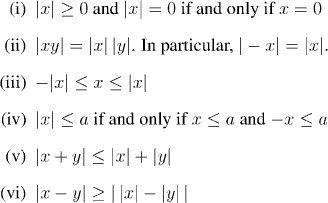## Open Sets of Real Numbers

Recall the notation: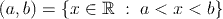for an open interval in the real line. The idea of open intervals is generalized in this section to an important class of subsets of the real line, called open sets. Open sets will be used a lot in Chapters 3, 5 and 6.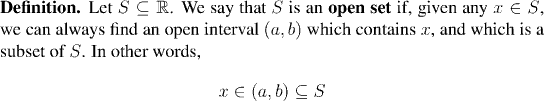Example:

Every open interval is an open set.

Example:

No finite subset of the real line is an open set.

Example:

The empty set is an open set.

Factoid:

The union of two open sets is also an open set.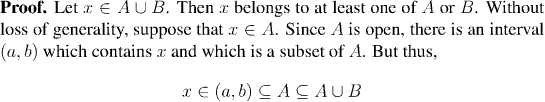Example: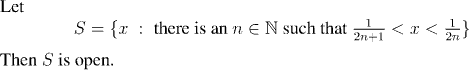Let's look at a picture of the set S: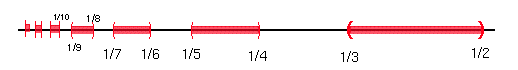From the picture it's clear that we can view S as a sort of union of the sets (1/(2n+1), 1/2n). But how can we talk about the union of infinitely many sets? (Note) In fact, taking the union (or intersection) of infinitely many sets will be important at many points through the course, so we now take a minute to give a careful definition of what it involves.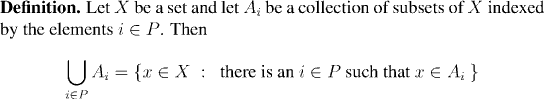Example: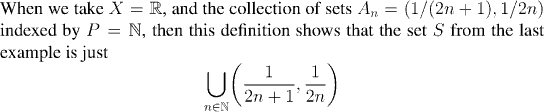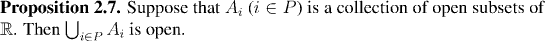Question: What do you think the definition of the intersection of an infinite collection of sets should be?

Question: The union of a family of open sets is open. Do you think the intersection of a family of open sets is also open?